# Space of path components

(diff) ← Older revision | Latest revision (diff) | Newer revision → (diff)
Jump to: navigation, search

## Definition

### As a set and topological space

Suppose$X$ is a topological space (we sometimes take a based topological space, but the basepoint turns out to be irrelevant). The space of path components of$X$, denoted$\pi_0(X)$, is defined as follows:

• As a set, it is the set of path components of$X$. In other words, it is the set of equivalence classes of$X$ under the equivalence relation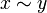$x \sim y$ iff there is a path from$x$ to$y$ in$X$.
• As a topological space, it is obtained by taking the quotient topology of$X$ under the equivalence relation$x \sim y$ iff there is a path from$x$ to$y$ in$X$.

For a locally path-connected space, the space of path components is a discrete space. Thus, often, when dealing with such spaces, we ignore the topology.

### As a monoid

If$X$ is a topological group, topological monoid, or (most generally) a H-space, then$\pi_0(X)$ naturally acquires the structure of a monoid (in fact, a topological monoid, but a discrete one if$X$ is locally path-connected). The structure is obtained by quotienting the original monoid (or H-space multiplication) by the equivalence relation of being path-connected.

In order to perform this quotienting, we need to justify that the relation of being in the same path component is a congruence for the original monoid (or H-space multiplication). This is indeed guaranteed by the continuity of multiplication: if$x_1$ and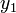$y_1$ are in the same path component, and$x_2$ and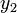$y_2$ are in the same path component, then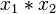$x_1 * x_2$ and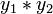$y_1 * y_2$ are in the same path component, because the paths can be multiplied pointwise.

Further, even if the original multiplication was not associative but only associative up to homotopy (making it an H-space), the new multiplication is strictly associative because any homotopy must descend to the identity map at the level of path components.